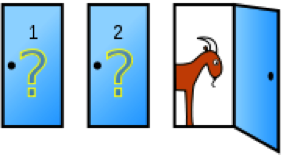# R Simulation – Monty Hall Problem

Suppose you’re on a game show, and you’re given the choice of three doors: Behind one door is a car; behind the others, goats. You pick a door, say No. 1, and the host, who knows what’s behind the doors, opens another door, say No. 3, which has a goat. He then says to you, “Do you want to pick door No. 2?” Is it to your advantage to switch your choice? (taken from Wikipedia)Shall I change doors or stick to my original choice? That is the question! Let’s write an R Script to run a simulation for this problem…

1. `# Monty Hall problem simulation`
2. ` `
3. `print("Monty Hall problem simulation")`
4. `print("-----------------------------")`
5. ` `
6. `# define variables`
7. `win.switch.count <- 0`
8. `win.noswitch.count <- 0`
9. `sim.count <- 50000`
10. `switch.count <- 0`
11. `noswitch.count <- 0`
12. ` `
13. `print(paste("Running", sim.count, "simulations"))`
14. ` `
15. `# run simulation for x times`
16. `for (i in 1:sim.count) {`
17. `    # create doors, a vector 1 to 3, for door 1, door 2, and door 3`
18. `    doors <- c(1, 2, 3)`
19. ` `
20. `    # set price behind a random door`
21. `    price.door <- sample(1:3, 1)`
22. ` `
23. `    # the guest picks up a random door`
24. `    guest.door <- sample(1:3, 1)`
25. ` `
26. `    # monty needs to open a door where there is a goat`
27. ` `
28. `    # monty door is not price and is not guest`
29. `    doors[price.door] <- 0`
30. `    doors[guest.door] <- 0`
31. ` `
32. `    # monty choose the max() door from the resultant vector`
33. `    monty.door <- max(doors)`
34. ` `
35. `    # re-insert the price door back in list!!`
36. `    doors[price.door] <- price.door`
37. ` `
38. `    # pick a number from 1 to 10 and if it is 5 or less, guest will switch door`
39. `    if (sample(1:10, 1) < 6) {`
40. `        # guest switch choice`
41. `        doors[monty.door] <- 0`
42. `        # since we are neutralising the vector and leaving only the only door not `
43. `        # chosen by the guest or monty`
44. `        # the sum of doors will be the door left`
45. `        guest.door <- sum(doors)`
46. `        switch.count <- switch.count + 1`
47. `        if (price.door == guest.door) {`
48. `            win.switch.count <- win.switch.count + 1`
49. `        }`
50. `    } else {`
51. `        # no switch`
52. `        noswitch.count <- noswitch.count + 1`
53. `        if (price.door == guest.door) {`
54. `            win.noswitch.count <- win.noswitch.count + 1`
55. `        }`
56. `    }`
57. `}`
58. ` `
59. `# display output`
60. `probability.switch <- win.switch.count / switch.count`
61. `probability.noswitch <- win.noswitch.count / noswitch.count`
62. `print(paste("P(switch door) =", probability.switch))`
63. `print(paste("P(no switch) =", probability.noswitch))`
64. `print(paste("Check P(switch) + P(no switch) =", probability.switch + probability.noswitch))`

Running the script returns something similar to:

``` "Monty Hall problem simulation"
 "-----------------------------"
 "Running 50000 simulations"
 "P(switch door) = 0.671743823458863"
 "P(no switch) = 0.332786360361803"
 "Check P(switch) + P(no switch) = 1.00453018382067"
>```

Switching increase the chance to win!

For a mathematical explanation we suggest to watch Numberphile’s video at https://www.youtube.com/watch?v=4Lb-6rxZxx0.